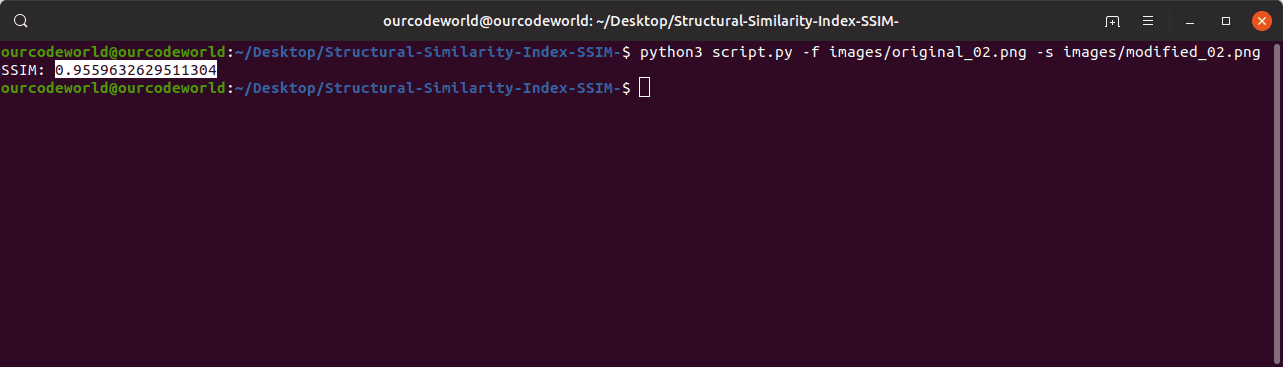# How to calculate the Structural Similarity Index (SSIM) between two images with Python

The Structural Similarity Index (SSIM) is a perceptual metric that quantifies the image quality degradation that is caused by processing such as data compression or by losses in data transmission. This metric is basically a full reference that requires 2 images from the same shot, this means 2 graphically identical images to the human eye. The second image generally is compressed or has a different quality, which is the goal of this index. SSIM is usually used in the video industry, but has as well a strong application in photography. SIM actually measures the perceptual difference between two similar images. It cannot judge which of the two is better: that must be inferred from knowing which is the original one and which has been exposed to additional processing such as compression or filters.

In this article, we will show you how to calculate this index between 2 images using Python.

## Requirements

To follow this tutorial you will need:

• Python 3
• PIP 3

Having said that, let's get started !

## 1. Install Python dependencies

Before implementing the logic, you will need to install some essential tools that will be used by the logic. This tools can be installed through PIP with the following command:

``pip3 install scikit-image opencv-python imutils``

These tools are:

• scikitimage: scikit-image is a collection of algorithms for image processing.
• opencv: OpenCV is a highly optimized library with focus on real-time applications.
• imutils: A series of convenience functions to make basic image processing functions such as translation, rotation, resizing, skeletonization, displaying Matplotlib images, sorting contours, detecting edges, and much more easier with OpenCV and both Python 2.7 and Python 3.

This tutorial will work on any platform where Python works (Ubuntu/Windows/Mac).

## 2. Write script

The logic to compare the images will be the following one. Using the `compare_ssim` method of the measure module of Skimage. This method computes the mean structural similarity index between two images. It receives as arguments:

#### X, Y: ndarray

Images of Any dimensionality.

#### win_size: int or None

The side-length of the sliding window used in comparison. Must be an odd value. If gaussian_weights is True, this is ignored and the window size will depend on sigma.

If True, also return the gradient with respect to Y.

#### data_rangefloat, optional

The data range of the input image (distance between minimum and maximum possible values). By default, this is estimated from the image data-type.

#### multichannelbool, optional

If True, treat the last dimension of the array as channels. Similarity calculations are done independently for each channel then averaged.

#### gaussian_weightsbool, optional

If True, each patch has its mean and variance spatially weighted by a normalized Gaussian kernel of width sigma=1.5.

#### fullbool, optional

If True, also return the full structural similarity image.

And returns:

#### mssimfloat

The mean structural similarity over the image.

The gradient of the structural similarity index between X and Y . This is only returned if gradient is set to True.

#### Sndarray

The full SSIM image. This is only returned if full is set to True.

As first, we will read the images with CV from the provided arguments and we'll apply a black and white filter (grayscale) and we'll apply the mentioned logic to those images. Create the following script namely `script.py` and paste the following logic on the file:

``````# Usage:
#
# python3 script.py --input original.png --output modified.png
# Based on: https://github.com/mostafaGwely/Structural-Similarity-Index-SSIM-

# 1. Import the necessary packages
from skimage.measure import compare_ssim
import argparse
import imutils
import cv2

# 2. Construct the argument parse and parse the arguments
ap = argparse.ArgumentParser()
ap.add_argument("-f", "--first", required=True, help="Directory of the image that will be compared")
ap.add_argument("-s", "--second", required=True, help="Directory of the image that will be used to compare")
args = vars(ap.parse_args())

# 3. Load the two input images

# 4. Convert the images to grayscale
grayA = cv2.cvtColor(imageA, cv2.COLOR_BGR2GRAY)
grayB = cv2.cvtColor(imageB, cv2.COLOR_BGR2GRAY)

# 5. Compute the Structural Similarity Index (SSIM) between the two
#    images, ensuring that the difference image is returned
(score, diff) = compare_ssim(grayA, grayB, full=True)
diff = (diff * 255).astype("uint8")

# 6. You can print only the score if you want
print("SSIM: {}".format(score))``````

This script is based on the code published by @mostafaGwely on this repository at Github. The code follows exactly the same logic declared on the repository, however it removes an error of printing the Thresh of the images. The output of running the script with the images using the following command:

``python3 script.py --first original_image.png --second compressed_image.png``

Will generate the following output (the command in the picture uses the short argument description `-f` as `--first` and `-s` as `--second`):The algorithm will print a string namely "SSIM: \$value", but you can change it as you want. If you compare 2 exact images, the value of SSIM should be obviously 1.0.

Happy coding !